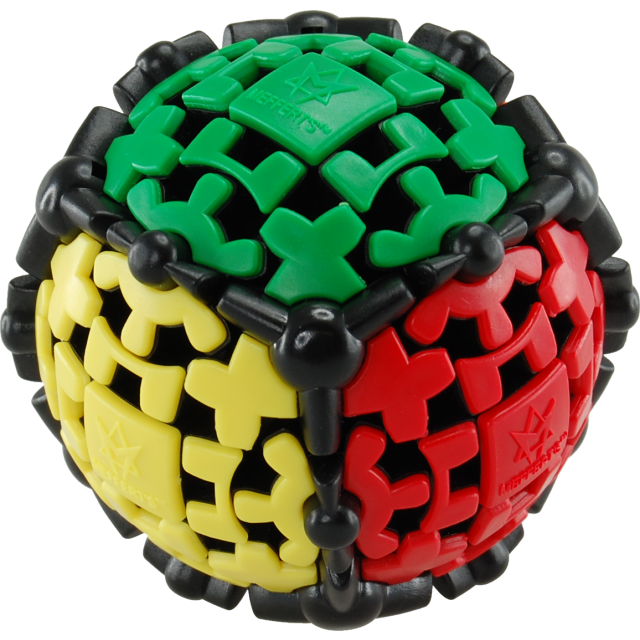# An Easy Solution for the Gear Ball Puzzle

My daughters keep bringing home various puzzle cubes, expecting me to solve them. The gear ball seems like an evil Rubik's cube. Rotating one face causes the opposing face to rotate in the opposite direction. At first my brain hurt watching it, but I now realize that the gears severely limit the number of possibilities. Here is a simple algorithm for solving it.Let R be a clockwise rotation of the right face and U a clockwise rotation of the upper face (with an opposite rotation of the L/D face due to the gears). R' and U' are the counterclockwise rotations.

I call the [ ] shaped pieces the “edges” and the + shaped pieces the “vertices”. The square pieces in the middle are the “faces”.

We need two moves that flip certain edges and vertices (between front/back, up/down, left/right):

```                               f/b       u/d       l/r

﹇        ﹇
R R U R R U'                            [   ]
﹈        ﹈

﹇+          +
R U R' U' R' U
+﹈         +
```
1. If any of the faces is surrounded by four differently colored edges, bring it to the front and do R U R' U'. You should now have at most two different edge colors around each face.
2. With ad-hoc moves, bring the yellow vertices around the yellow face.
3. Using the first move (R R U R R U'), fix the yellow edges (appropriately rotating the ball).
4. If two of the vertices surrounding the blue face are green, do the second move with the yellow face in front.
5. If four of the vertices surrounding the blue face are green, put the blue face in front and rotate the yellow face 180 degrees so that the blue face has four blue vertices.
6. Using the first move again, fix the blue edges while keeping yellow to the right.
7. Fix the wheels by rotating each face 360 degrees until they are in order.
8. You are done.